### Group Theory

Welcome to the beautiful and useful world of group theory! Groups are one of the cornerstones of modern mathematics, appearing in many places, including:

• the mathematical description of symmetry
• the structure of the Rubik’s Cube and how to solve it
• simple solutions to otherwise difficult combinatorics problems
• the definition of the determinant of a matrix
• cryptographic systems, distinguishing topological shapes from each other, and much more.

But we’ll start off simply: by looking at the symmetries of a triangle.

# Symmetry

A rigid transformation of an equilateral triangle maps the triangle back to itself. Here’s what that means, intuitively: imagine that the triangle is made of rigid plastic, and you put it on a piece of paper and draw its outline with a pen. A rigid transformation is a way of picking the triangle up, moving it around without bending or tearing it, and putting it back down so that it fits inside the outline you drew.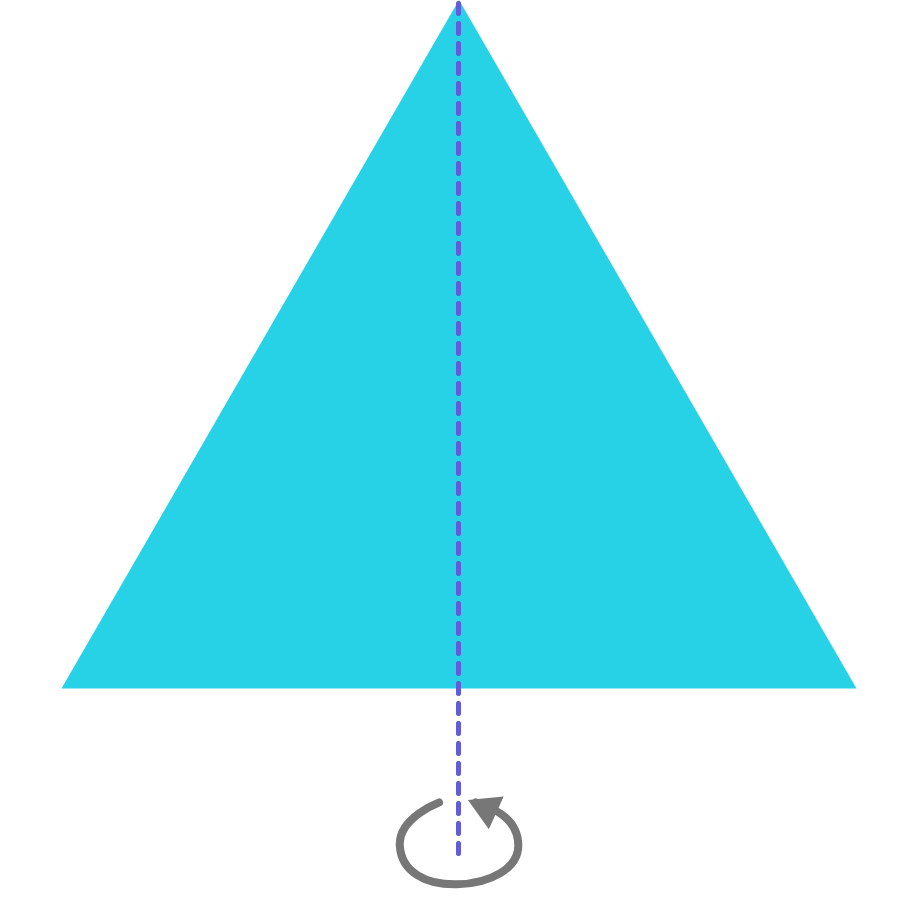One such transformation is rotating the triangle clockwise by $240\degree,$ which we consider equivalent to rotating the triangle counterclockwise by $120\degree,$ since both leave the vertices of the triangle in identical positions. Another is reflecting the triangle about a line. It's also easy to forget the transformation defined by not changing the position of the triangle's vertices at all, which we will call the identity transformation.

How many rigid transformations does an equilateral triangle have? (Do not count transformations separately if they are equivalent.)

# Symmetry

What is the connection with symmetry? Well, when you casually say a shape has symmetry, like the capital letter A, that just means there’s a rigid transformation, horizontal reflection, that sends A to itself. The letter H has at least one more symmetry, because now there is another rigid transformation that sends it to itself, vertical reflection. We will define a symmetry of an object to be a rigid transformation from that object to itself.

So, from the last problem, we will say an equilateral triangle has six symmetries: three rotations and three reflections.

Two more points: Since symmetries are rigid transformations, they are really functions. We only care about where the points end up, not how they got there. In the example of the triangle, rotating clockwise by $120\hspace{0.3mm}^{\circ}$ is considered the same symmetry as rotating clockwise by $480\hspace{0.3mm}^{\circ}$ $($i.e. $360\hspace{0.3mm}^{\circ} + 120\hspace{0.3mm}^{\circ}),$ and the same symmetry as rotating counter-clockwise by $240\hspace{0.3mm}^{\circ}.$

Also, we will always count the “identity symmetry,” corresponding to picking up the triangle, not changing it, and putting it right back down. This corresponds to the identity rigid transformation $f(x) = x.$

# Symmetry

How many rotation and reflection symmetries are there in a square? (Again, remember to include the identity symmetry.)

Note: We only care about where the points end up, not how they got there.# Symmetry

Let’s look at something a little more complicated. Consider the modified square shown below.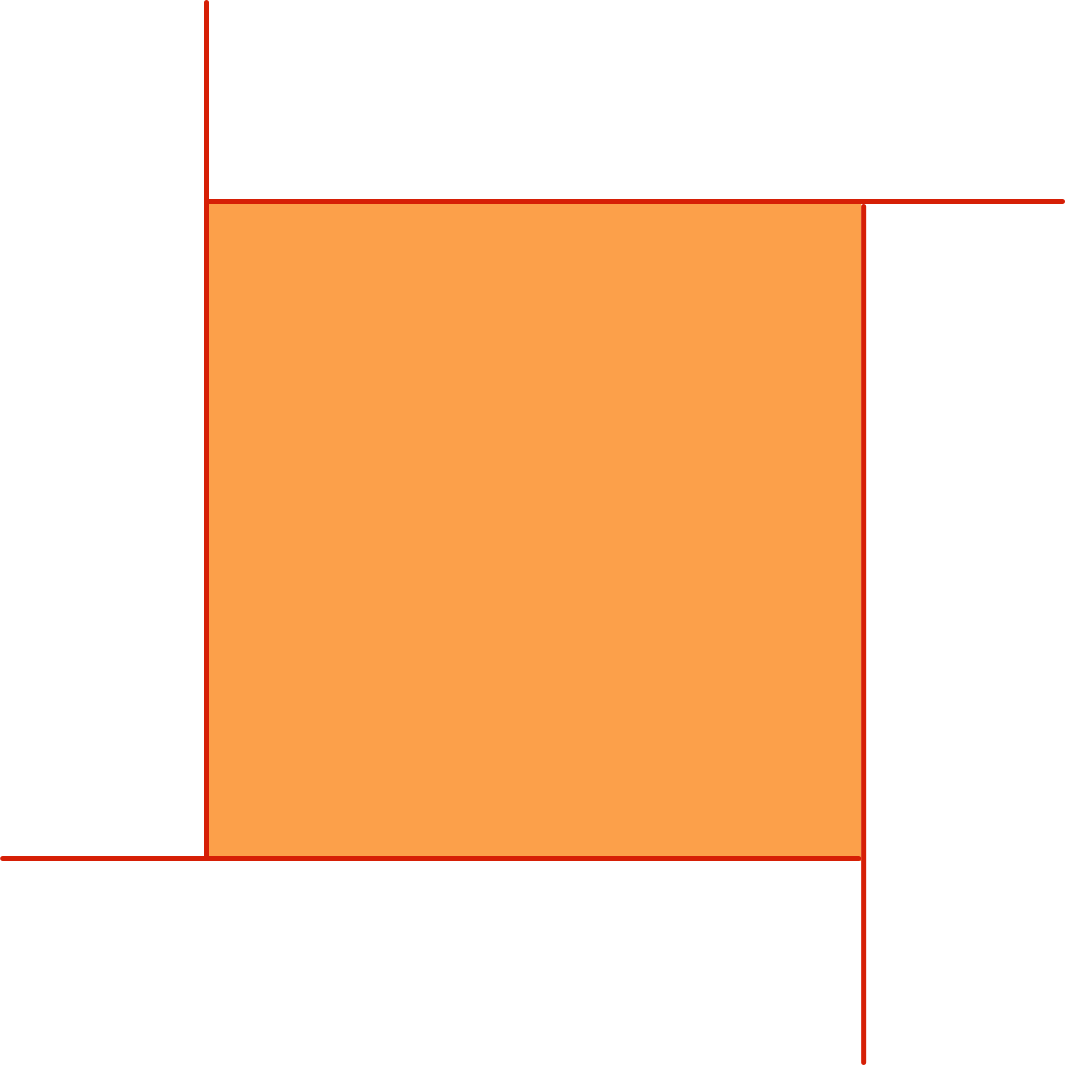How many of the $8$ symmetries of the regular square found in the last problem reproduce the figure above exactly?

# Symmetry

One more example. Every shape has the identity transformation for a symmetry. The most asymmetric a shape can be is to have no other symmetries, like the letter Q.

Consider all the capital letters shown below (in the exact font they are in). How many of them have only the identity symmetry?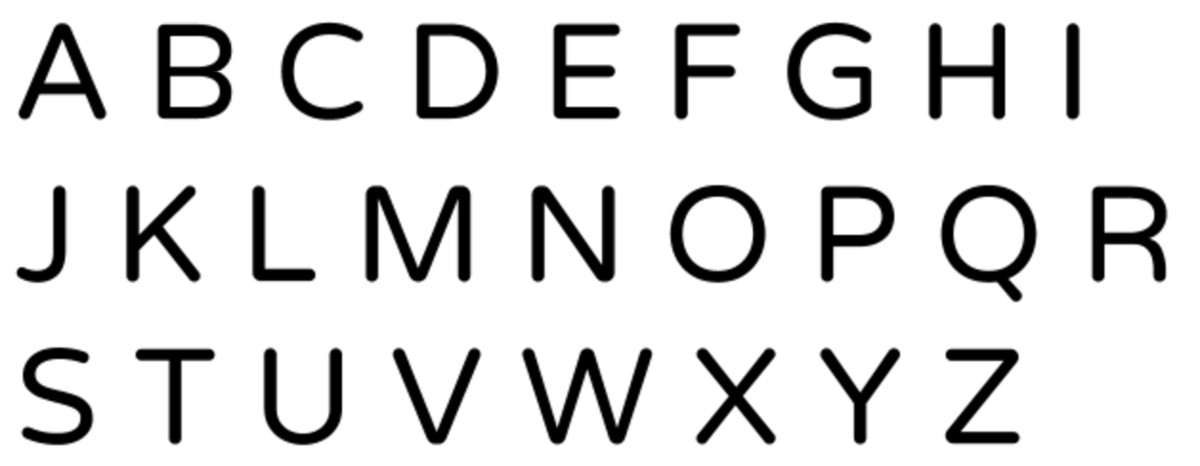# Symmetry

To recap, every object has a set of symmetries, which are the rigid transformations sending the object to itself.

We've looked at triangles, squares and letters. There are other interesting shapes to consider. How many symmetries are there in a circle? (Hint: a lot!) Also, there’s no reason to restrict ourselves to two dimensions. Later on, we’ll consider the symmetries of three-dimensional objects like tetrahedra and cubes.

Now, the more symmetries an object has, the more “symmetric” we think it is. But there’s more to an object’s symmetry than just the number of its symmetries, as the next example will show. This is why we can’t just use a number to fully describe how symmetric the object is. We will need a more complicated object to measure symmetry: the group.

# Symmetry

Which of these two objects has more symmetries?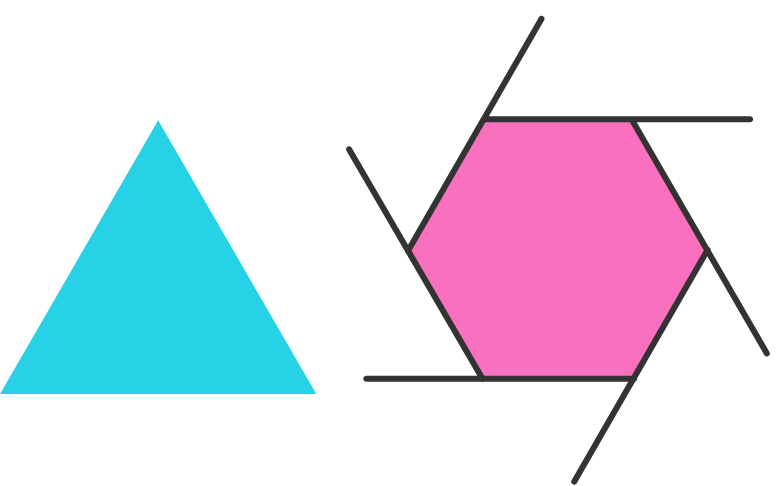# Symmetry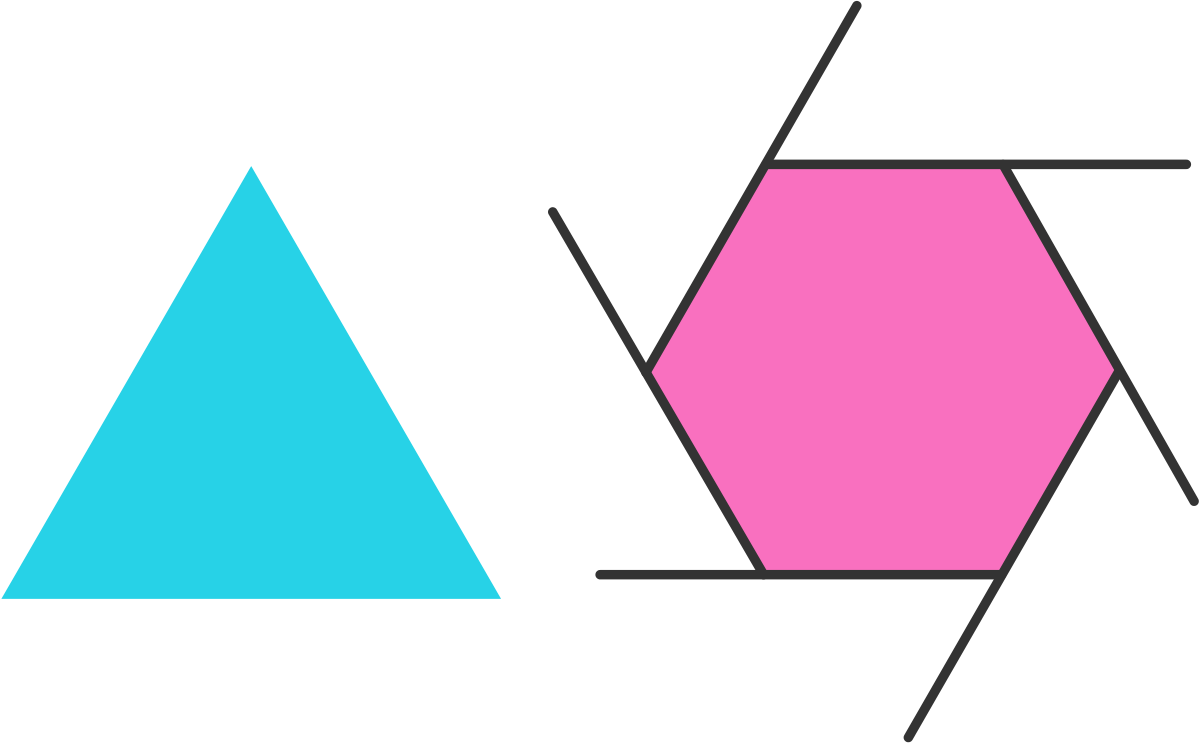The two objects from the last problem each have six symmetries specific to their respective shapes (assuming the vertices are labeled); despite their superficial similarities, there's something fundamentally different about the two collections of symmetries.

The difference will involve the idea of doing a transformation multiple times in a row. Notice that if you do a reflection twice in a row, you get back to where you started. We might say that when you do it twice, you get the identity transformation. Similarly, if you rotate the triangle by $120\hspace{0.3mm}^{\circ}$ three times, you get the identity transformation.

Which of the following statements that distinguish the two sets of symmetries is true?

A. The triangle has a symmetry with the property that when you apply it twice in a row, you get the identity transformation, whereas the hexagon has no such symmetry.

B. The triangle has a symmetry with the property that when you apply it three times in a row, you get the identity transformation, whereas the hexagon has no such symmetry.

C. The hexagon has a symmetry with the property that when you apply it less than six times, you don’t get the identity transformation, but when you apply it six times you do, whereas the triangle has no such symmetry.

# Symmetry

Group theory is an area of algebra, which means that we will be studying how combining objects can make new objects. That’s what started to happen in the last problem, when we combined symmetries with themselves. To use notation we will explore in the next quiz, the correct answer to that problem (C) can be rephrased as follows: Only the hexagon has a symmetry $S$ with the property that $S \neq I,$ $S^2 \neq I,$ $S^3 \neq I,$ $S^4 \neq I,$ and $S^5 \neq I,$ but $S^6 = I,$ where $I$ is the identity transformation.

It looks like we’re starting to develop an algebraic system that looks like multiplication. Take the next quiz to see more!

# Symmetry

×